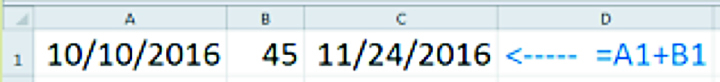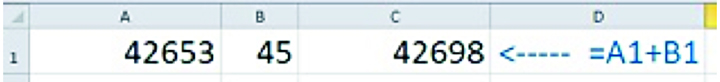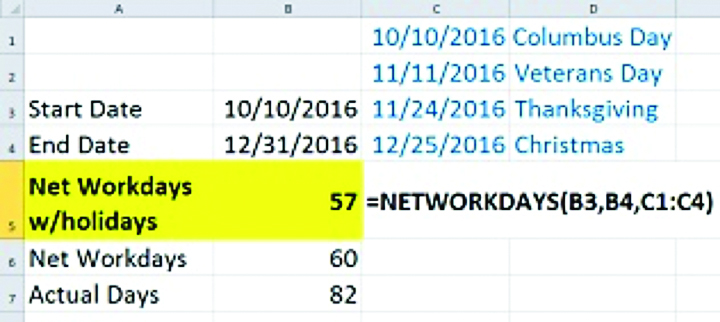# Excel: Date data demystified

There’s something at which Excel truly excels that surprises even many advanced users, and that’s date math. Once you know how Excel works with dates, there’s so much your data can tell you!

Serial number dates

When Excel stores a date, although you may see it as 10/10/2016, it is actually storing the number 42,653. Because with date formatting, the number “1” comes out to be 1/1/1900, so 42,653 days later, it’s 10/10/2016. So, if you’re solving for a future date, you simply add the number of days to the starting date to find out what date that would be. For example, if you enter the date a factory floor machine is put into operation in A1, the number of days after which it must be recalibrated in B1, and the formula =A1+B1 in C1, you would get the correct date.From Excel 2007 through the current versions, if you type in the date + some number, you will get a date in return. But, occasionally when doing math with dates, you’ll get the number in return. Either way, the result is correct, but the formatting may need to be adjusted.Consider this: If you typed the formula in this way, you have typed in the exact same values as the date-formatted one above it. You just formatted the numbers differently. So if you were expecting a date, just format it as one.

Tip: To quickly enter today’s date use Ctrl+; (semicolon).

Date functions

There are functions you can use in Excel with dates that help you calculate date spans and pull specific date elements out of a correctly formatted date. You can even take an incorrectly formatted date and correct it with date functions.

First, the basics. TODAY() and NOW() will both return the date your computer thinks it is today. The NOW() variation will also return the time. If you always want to know the relationship between the due date of an invoice and today’s date, the formula would be =TODAY()-. If the result is 0, the due date is today. If the date is negative, the invoice isn’t due yet. If it’s a positive number, the invoice is past due by that number of days. When you’re having trouble remembering what to put where, it helps to remember that “later than is greater than.”

If you need to pull just the year, month or day value out of date, you can use the functions, YEAR(), MONTH(), or DAY(). IF were 10/10/2016, these would result in 2016, 10, and 10, respectively.

Need to eliminate weekends and holidays? Try NETWORKDAYS(,,). The holidays argument is optional.But consider these differences in calculating the number of days left in 2016, if you start counting from 10/10/2016.

Some gotchas